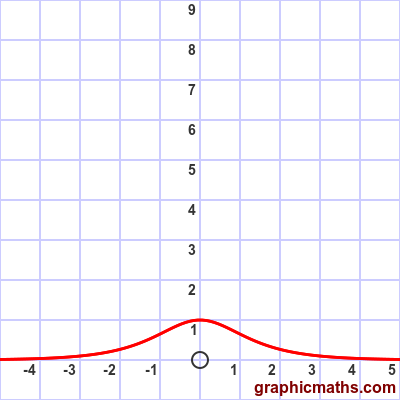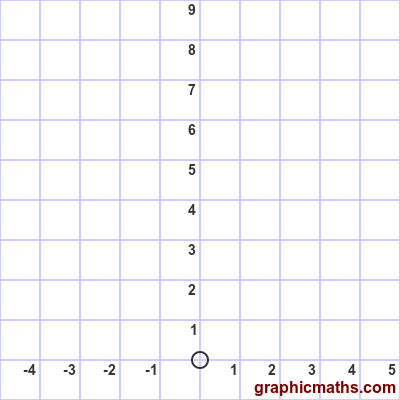# sech function

By Martin McBride, 2021-02-04
Tags: sech cosh inverse hyperbolic function
Categories: hyperbolic functions pure mathematics

The sech function is a hyperbolic function. It is also known as the hyperbolic secant function.

Here is a video that explains the cosech, sech, and coth functions:

## Equation and graph

The sech function is the reciprocal of the cosh function:

$$\operatorname{sech}{x} = \frac{1}{cosh{x}}$$

(This is analogous with trig functions, where sec is the reciprocal of cos.)

Substituting the formula for cosh gives:

$$\operatorname{sech}{x} = \frac{2}{e^{x}+e^{-x}}$$

Here is a graph of the function:## Sketching sech

We can sketch the sech curve based on the cosh curve:The cosh curve tends to +∞ for large negative $x$, it has a value of 1 when $x = 0$, and it tends to +∞ for large positive $x$.

The sech curve is the reciprocal of cosh. It tends to zero for large negative $x$, it has a value of 1 when $x = 0$, and it tends to +∞ for large positive $x$.

## Other forms of the equation

If we take the two alternative forms of the cosh function, and invert them, we get two alternative forms of the sech function:

$$\operatorname{sech}{x} = \frac{2e^{x}}{e^{2x}+1}$$

$$\operatorname{sech}{x} = \frac{2e^{-x}}{1+e^{-2x}}$$